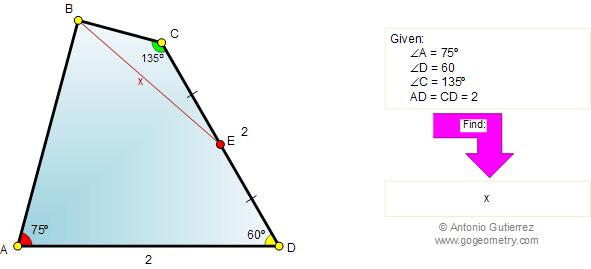Problem 405. Quadrilateral, 60, 75, and 135 degrees, Midpoint. Level: High School, College, SAT Prep. The figure shows a quadrilateral ABCD with AD = CD = 2, and the measure of the angles A, C, and D are equal to 75, 135, and 60 degrees, respectively. If E is the midpoint of CD, find BE.Recent Additions